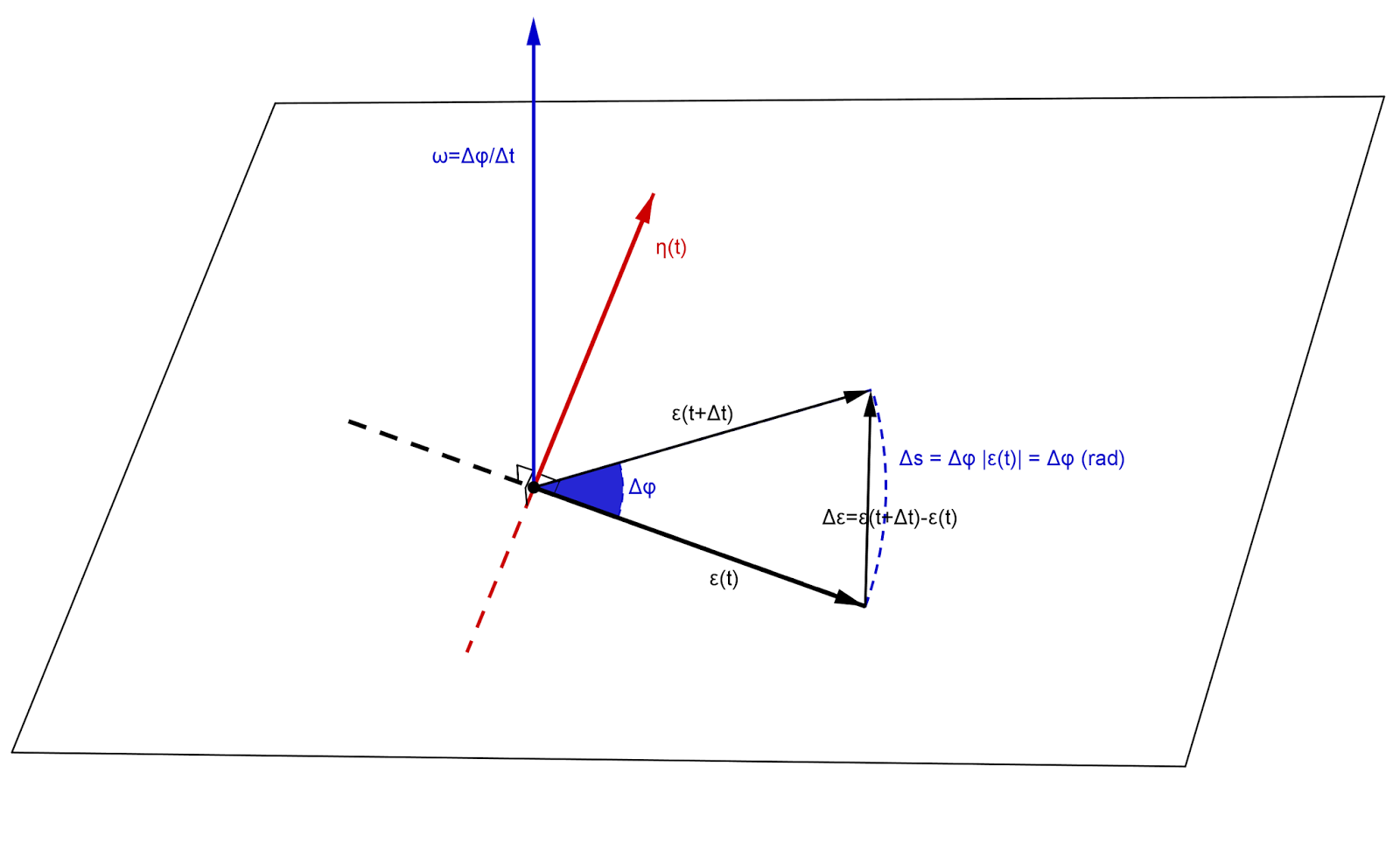## Monday, January 20, 2014

### Derivative of a unit vector

In today's post I would like to present a simple but elegant construction: the computation of the derivative of a rotating unit vector i.e. of a vector function $\vec{\varepsilon}: [0, +\infty) \rightarrow \mathbb{R}^{2}$ whose length is equal to one $|\vec{\varepsilon}(t)|=1$ for any $t \in [0, +\infty)$. I will use $t$ to denote the independent variable since it normally stands for time. Thus, at any given instant $t$, the value of the function will be a  unit vector $\vec{\varepsilon}(t)$.
We will apply the definition, so we need to compute the vector
\begin{equation} \label{def1}
\frac{d}{dt}\vec{\varepsilon}(t) = \lim_{\Delta t \rightarrow 0} \frac{\vec{\varepsilon}(t + \Delta t) - \vec{\varepsilon}(t)}{\Delta t}
\end{equation}
First note that since $|\vec{\varepsilon}(t)|^{2} = \vec{\varepsilon}(t) \cdot \vec{\varepsilon}(t) = 1$ by differentiating both sides we get
$$\begin{array}{c} \frac{d}{dt} \big( \vec{\varepsilon}(t) \cdot \vec{\varepsilon}(t) \big) = \frac{d \vec{\varepsilon}(t)}{dt} \cdot \vec{\varepsilon}(t) + \vec{\varepsilon}(t) \cdot \frac{d \vec{\varepsilon}(t)}{dt} = \\ \\ 2 \frac{d \vec{\varepsilon}(t)}{dt} \cdot \vec{\varepsilon}(t) = 0 \Leftrightarrow 2 \frac{d \vec{\varepsilon}(t)}{dt} \cdot \vec{\varepsilon}(t) = 0 \end{array}$$
Thus $\frac{d \vec{\varepsilon}(t)}{dt} \cdot \vec{\varepsilon}(t) = 0$ which is equivalent to the fact that $\vec{\varepsilon}(t)$ and its derivative vector $\frac{d \vec{\varepsilon}(t)}{dt}$ are perpendicular
\begin{equation} \label{def2}
\vec{\varepsilon}(t) \bot \frac{d \vec{\varepsilon}(t)}{dt}
\end{equation}
The situation can be presented in the following picture:$\Delta s$ is the length of the arc spanned by the edge of the unit vector $\vec{\varepsilon}(t)$ while it rotates by an angle of $\Delta \varphi$ during time $\Delta t$. Since $\varphi$   is measured in radians we have $\Delta s = \Delta \varphi |\vec{\varepsilon}(t)|$ which implies that
$$\Delta s = \Delta \varphi$$

Notice that (in the figure) we also have:  $|\vec{\eta}(t)|=1$ and $\ \vec{\eta}(t) \bot \vec{\varepsilon}(t)$.
Now, during that time interval $\Delta t$ the vector has changed by
$$\Delta \vec{\varepsilon} = \vec{\varepsilon}(t + \Delta t) - \vec{\varepsilon}(t)$$
While $\Delta t \rightarrow 0$ (the notation $dt \rightarrow 0$ will be used instead from now on) we can notice two things: i). the direction of the vector $d \vec{\varepsilon} = \vec{\varepsilon}(t + dt) - \vec{\varepsilon}(t)$ "tends" to become perpendicular to $\vec{\varepsilon}(t)$ and thus parallel to the direction specified by $\vec{\eta}(t)$ and ii). the length of the vector $d \vec{\varepsilon}$ "tends" to become equal to the length of the arc $ds$ thus
\begin{equation}  \label{def3}
|d \vec{\varepsilon}| = |\vec{\varepsilon}(t + dt) - \vec{\varepsilon}(t)| = ds = d \varphi
\end{equation}
(where $d \varphi$ is measured in radians).
Now, combining \eqref{def2} and \eqref{def3} we can write \eqref{def1} as follows
$$\begin{array}{c} \frac{d}{dt}\vec{\varepsilon}(t) = \lim_{dt \rightarrow 0} \frac{\vec{\varepsilon}(t + dt) - \vec{\varepsilon}(t)}{dt} = \lim_{dt \rightarrow 0} \frac{d \varepsilon}{dt} = \frac{d \varphi}{dt} \vec{\eta}(t) \end{array}$$
Finally, if we define a vector $\vec{\omega}$ with direction perpendicular to the plane defined by $(\vec{\varepsilon}(t), \vec{\eta}(t))$ and with length $|\vec{\omega}|=\frac{d \varphi}{dt}$ then we have
$$\frac{d}{dt}\vec{\varepsilon}(t) = \vec{\omega} \times \vec{\varepsilon}(t) = \frac{d \varphi}{dt} \vec{\eta}(t)$$